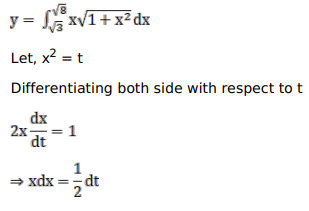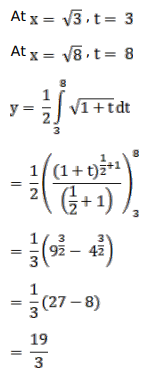# Mark against the correct answer in the following:

Question:

Mark $(\sqrt{)}$ against the correct answer in the following:

$\int_{\sqrt{3}}^{\sqrt{8}} x \sqrt{1+x^{2}} d x=?$

A. $\frac{19}{3}$

B. $\frac{19}{6}$

C. $\frac{38}{3}$

D. $\frac{9}{4}$

Solution: